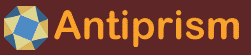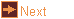# EXTRA: sweep_edges - sweep edges to make a surface

 Usage    |    Examples    |    Notes

## Usage

```

Usage: sweep [options] input

Read input model in OFF format and transform it multiple times to produce
a polygonal surface made of the quadrilaterals swept by the edges. At
each step the input model is transformed according to the specified
transformations, which can be defined using sweep progress variables FRAC
and ANG. FRAC runs from 0.0 at at step 0 to 1.0 on the final step.
FRAC = current_step / total_steps
ANG = 360.0 * FRAC
Colours are taken from the input model if the begining of the sweep does
not coincide with the end, or if option -O is set, otherwise sweep circuits
are found and the colours are set from the colour map (option -m). Vertex
colours are used for final vertices and swept vertex edges, edge colours
are used for swept edge faces.
If input is not given the program reads from standard input.

Options
-h,--help this help message (run 'off_util -H help' for general help)
--version version information
-n <int>  number of steps (default: 12)
-T <tran> translate, three numbers separated by commas which are
used as the x, y and z displacements
-R <rot>  rotate about an axis, three, four or six numbers separated by
commas. If three numbers these are angles (degrees) to rotate
about the x, y and z axes. If four numbers, the first three
are a direction vector for the axis, the last number is the
angle (degrees) to rotate. If six numbers, these are two
vectors (from,to) and rotate to carry the first to the second.
If twelve numbers these are four vectors (from1,from2,to1,to2)
and rotate to carry the first onto the third then rotate around
the third to carry the second onto the fourth
-M <norm> reflect in a plane, three numbers separated by commas which
give a vector normal to the plane of reflection.
-S <scal> scale, one, three or four numbers separated by commas. If one
number then scale by this factor in all directions. If three
numbers these are the factors to scale along the x, y and
z axes. If four numbers, the first three are a direction
vector for the scaling, the last number is the factor to scale
-I        inversion
-A <crds> transformation that will align two sets of three points
(18 numbers coordinates of from1,from2,from3,to1,to2,to3)
-a <angs> transformation that makes particular angles between the
mapped axes, angles in degrees in form yz_ang,zx_ang,xy_ang
(corresponding to the angles opposite the x-, y- and z-axis)
-X <mtrx> transformation matrix of 9 or 12 values, given left-to-right
top-to-bottom, used to premultipy each coordinate
-i        replace the current combined transformation by its inverse
-t        triangulate
-O        do not join coincident sweep ends
-l <lim>  maximum distance between vertices that should be coincident, lim
is an integer represeting the negative exponent of the distance
(default: 8 giving 1e-08)
-m <maps> a comma separated list of colour maps used to transform colour
indexes (default: rand), a part consisting of letters from
v, e, f, selects the element types to apply the map list to
(default 'vef'). The 'compound' map should give useful results.
-o <file> write output to file (default: write to standard output)
```

## Examples

Torus sweep. Using the hexagon model (with coordinates in the xy-plane), translate it 2 units along the x-axis (-T 2,0,0), progressively rotate it 1 turn around the y-axis (-R 0,ANG,0)

```sweep_edges pol6 -T 2,0,0 -R 0,ANG,0 | antiview
```

Twisted torus sweep. Using the hexagon model, progressively rotate it two edges around the z-axis (-R 0,0,ANG*2/6), translate it 2 units along the x-axis (-T 2,0,0), progressively rotate it 1 turn around the y-axis (-R 0,ANG,0). Sweep using 30 steps. Displaying the model with antiview -t no_tri -x ev produces a smoother look to the surface and hides vertices and eges.
```sweep_edges pol6 -n 30 -R 0,0,ANG*2/6 -T 2,0,0 -R 0,ANG,0 | antiview -t no_tri -x ev
```

Twisted torus sweep. As before, but turn a nonagon 6 edges while sweeping, and sweep at a larger radius. Setting the vertex and edge colours from a different colour map and then displaying the models with antiview -t no_tri -v 0.05 -e 0.05 -E invisible makes a smoother surface and shows the paths of the original vertices.
```sweep_edges pol9 -n 30 -R 0,0,ANG*6/9 -T 4,0,0 -R 0,ANG,0 -m spread+3,ve | antiview -t no_tri -v 0.1 -e 0.1 -E invisible
```

Twisted double wound torus sweep. Using the triangle model, translate it 0.5 units on the x-axis (-T 0.5,0,0), progressively rotate it one turn around the z-axis (-R 0,0,ANG), translate it 2 units along the x-axis (-T 2,0,0), progressively rotate it 2 turns around the y-axis (-R 0,2*ANG,0).
```sweep_edges -n 200 -T 0.5,0,0 -R 0,0,ANG -T 2,0,0 -R 0,2*ANG,0 pol3 | antiview -t no_tri -x ev
```

Tapered helix sweep. Colour the edges of a triangle model, scale it by 0.5 (-S 0.5), progressively translate it 2 to 1 units on the x-axis (-T "2*(1-FRAC),0,0"), progressively translate it 5 to 0 units on the y-axis (-T 0,5*FRAC,0), progressively rotate it 10 turns around the y-axis (-R 0,10*ANG,0). Note that maths expressions using special characters lie '(' may need the option argument to be surrounded by quotes, or escaped in some other way.
```off_color -e U pol3 | sweep_edges -n 200 -S 0.5  -T "2*(1-FRAC),0,0" -T 0,5*FRAC,0 -R 0,10*ANG,0 | antiview -t no_tri -x ev
```

Incomplete dipyramid sweep, which is the repeated symmetrically. Make a couple of coloured edges with vertices on the y-axis. Sweep 3/4 of a turn around the y-axis in 12 steps (to make a 16-gon dipyramid with a 1/4 missing). In the second command remake the model and repeat it symmetrically to be aligned with the edges of a cube.
```off_util null -Av0,1,0 -Av1,0,0 -Av0,-1,0 -Ae0,1:red -Ae1,2:green | sweep_edges -n 12 -R 0,3*ANG/4,0 | antiview -t no_tri
off_util null -Av0,1,0 -Av1,0,0 -Av0,-1,0 -Ae0,1:red -Ae1,2:green | sweep_edges -n 12 -R 0,3*ANG/4,0 | off_trans -T -1,0,-1 | poly_kscope -s T | antiview -t no_tri -x ev
```

Klein bottle
```sweep_edges pol4 -R 0,ANG/2,0 -T "sin(ANG/2),0,0" -R 0,ANG,0 -n 200 | off_util -M a | antiview -t no_tri -x ev
```

Making a twisted surface with "sides" made from sweeping multiple edges may benefit from a specified colouring. The following commands show
• a twisted square torus
• a similar model but the sides are made from several edges
• an open tube model is made with -O, that preserves the original colours, and colours mismatch
• an open tube model is made with -O, and the original colour indices are mapped so they match

```sweep_edges -n 64 -R 0,0,2*ANG/4 -T 3,0,0 -R 0,ANG,0 -m map_red:green pol4 | antiview -t no_tri -x ev
off_util -g -s pol24 -Dv7- | off_trans -T -3.830648787770195,3.830648787770195,0 | poly_kscope -s C4 -c e | off_util -M a | sweep_edges -n 64 -R 0,0,2*ANG/4 -T 10,0,0 -R 0,ANG,0 | antiview  -t no_tri -x ev
off_util -g -s pol24 -Dv7- | off_trans -T -3.830648787770195,3.830648787770195,0 | poly_kscope -s C4 -c e | off_util -M a | sweep_edges -n 64 -R 0,0,2*ANG/4 -T 10,0,0 -R 0,ANG,0 -O | antiview  -t no_tri -x ev
off_util -g -s pol24 -Dv7- | off_trans -T -3.830648787770195,3.830648787770195,0 | poly_kscope -s C4 -c e | off_util -M a | sweep_edges -n 64 -R 0,0,2*ANG/4 -T 10,0,0 -R 0,ANG,0 -O -m map_red:green:green:red | antiview  -t no_tri -x ev
```

Plane tiling with progressing scaling
```off_util null -Av2,0,0 -Av3,0,0 -Ae0,1 | sweep_edges -n 80 -R 0,0,5*ANG -S "((2/3)^(5*FRAC))"| off_util -l 10 -M a  | wythoff -c j | off_color -f P -m map_green:brown| antiview
```

## Notes

Swept surfaces are sometimmes used in sculpture, for example Enrique Carbajal (Sebastian) and Helaman Ferguson. The n_icons program sweeps half a polygon twice, as a progressive half turn, a twist, then another progressive half turn.